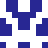### Real Vim ninjas count every keystroke - do you?

###### Pick a challenge, fire up Vim, and show us what you got.

```Your VimGolf key: please sign in

\$ gem install vimgolf
\$ vimgolf setup
```

### Simple format (2)

try again!

##### Start file
```a==b equal to
a!=b not equal to
a>b greater than
a>=b greater than or equal to
a<b less than
a<=b less than or equal to
```
##### End file
```	a == b		equal to
a != b		not equal to
a >  b		greater than
a >= b		greater than or equal to
a <  b		less than
a <= b		less than or equal to
```

#### View Diff

```1,6c1,6
< a==b equal to
< a!=b not equal to
< a>b greater than
< a>=b greater than or equal to
< a<b less than
< a<=b less than or equal to
---
> 	a == b		equal to
> 	a != b		not equal to
> 	a >  b		greater than
> 	a >= b		greater than or equal to
> 	a <  b		less than
> 	a <= b		less than or equal to
```

### Solutions by @rike09:

Unlock 5 remaining solutions by signing in and submitting your own entry

## 68 active golfers, 328 entries

##### Solutions by @rike09:29
###### #31 - Henrique Malheiro / @rike09

11/22/2018 at 12:50AM30
###### #>33 - Henrique Malheiro / @rike09

11/22/2018 at 12:41AM32
###### #>39 - Henrique Malheiro / @rike09

11/22/2018 at 12:34AM34
###### #>45 - Henrique Malheiro / @rike09

11/22/2018 at 12:33AM39
###### #>58 - Henrique Malheiro / @rike09

11/22/2018 at 12:25AM